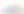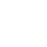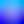August 10, 2021

# Intro to Pandas: how to manipulate Data in PythonIronhack - Changing The Future of Tech Education

Data Analytics

All CoursesOrganising data is essential when it comes to data analysis, and this process is called data manipulation. It is a crucial step in data analytics. Whether you need to represent information using a graph, combine multiple datasets, create a pivot table or change an Excel file to a CSV file, Pandas is the best Python library for the task. The Pandas library was written specifically for the Python programming languages, and aside from creating graphs, it lets you arrange data and perform other functions. These include merging data sets, reading records, grouping data and organising information in a way that best supports the analysis required. It is a straightforward, accessible and versatile library that is suitable for new and experienced developers alike.

## Pandas Library

“Pandas” stands for Python Data Analysis Library. There is a multitude of ways to work with data in Python. Depending on how you wish to manipulate your data, you generally need to follow a few coding simple steps and select the relevant syntax in the overall code. For starters, however, Pandas needs to be installed in order to avail of it. It is available across all systems, Windows, Mac OS and Linux, but note that it is dependent on the NumPy Python library, plus, it may require additional libraries depending on the tasks you need to perform. For plotting, for example, Matplotlib will be required.

### Representing Data Using Pandas

If you wish to represent numerical information in a line chart, bar graph, pie chart or scatter diagram, for example, you would simply follow these steps using code from the Python Pandas library:

• Prepare the data: this could be done by entering it into a simple table or Excel sheet

• Create a DataFrame by running a code in Python

• Plot the DataFrame using the relevant syntax: in this step you can specify the type of chart by using the code kind = ‘xxx’ (e.g. kind = "line" would create a line chart.) The Matplotlib syntax will be needed in this step

• Run the code and watch your data come to life in a chart or graph

## Pandas, Data & Matplotlib

The complete Python codes can be found online and in the Matolib library, but to change the type of graph you are creating simply use the relevant “kind” code. Kind = “bar” would create a bar chart, while kind = “scatter” would create a scatter diagram.

### Merging Data with Python

Another type of data manipulation that can be performed using Pandas is merging datasets. Let’s say you have 2 sets of data that need to be combined. You can follow these steps to join or merge them:

• Prepare the data: if you have two datasets, then you will have two separate tables to start with

• Create two DataFrames using the Python code

• Merge the Pandas DataFrames using a join code

• Run the code to view the results

## Create Two DataFrames Using the Python Code

There are various codes for combining data in Pandas DataFrames, depending on where you are taking the information from and how you wish to combine it. For instance, you can use the merge function - merge( ) -for merging data on a common column, while the .join( ) code will let you combine data on a specific column.

### Create a Pivot Table

Another very popular form of data manipulation is creating a pivot table. Pivot tables can be generated with Microsoft Excel or spreadsheets, though it is also possible to create them easily with Python. Pivot tables are used to reorganise, sort or summarise data, and let you create an overview of information in any way you wish.

Depending on what you need to use a pivot table for, you can select the most appropriate Pandas code for the job. You might need to manipulate data to determine the total number of emails sent to one company by a team over the course of a month, for example, or find the median sales for Q1 in a given location. Begin by again, preparing the data in a simple table and capturing it in Python by running a DataFrame code. Depending on your goal, you can then use the relevant Python syntax in the code in order to produce the pivot table.

### Pivot Table to Graph

To go one step further with Pandas, data and results from a pivot table can be represented in a graph or chart, as outlined above. For this, you would just need to add some additional components to the Pivot Table code.

## Calculating Stats from a CSV file

Statical analysis is another area where Pandas, data manipulation and python are regularly used. If you create a file using Python, it is possible to use the Pandas library to calculate stats - this may be to find the median salary across an entire company, for example, or to measure the standard deviation of salaries among different teams. First, copy your dataset into a CSV file and import it into Python using a code template. Next, run a code to calculate the statistics. Once you run the relevant code, you will generate a summary of the desired results.

### Data Analytics Course

These are just a few of the options when it comes to manipulating data with Python. The Pandas library gives you a huge amount of control and flexibility over your data and lets you represent it very specifically. Once you understand the basics of data manipulation with Python, it is easy to build on that knowledge and use the library for lots of different analytical and representational tasks. Get started with Python and the fundamentals of data analytics with the Data Analytics Bootcamp. If you wish to acquire skills in Pandas, Data Analytics and Python, along with Git and SQL, an online course is a great place to start. Pandas, data and the Python coding language go hand in hand, and anyone working in web development, data or statistical analysis would be very well equipped with this skillset under their belt. It is also very useful for careers in sales, business development and digital marketing; it lets you work flexibly with numbers and also strengthens reporting capabilities.